Error Plotting EquationClassicListThreaded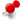4 messagesOpen this post in threaded view
|

Error Plotting Equation

 I have tried to plot equation in RC 2.2.0 RC1. It is done from menu "Plugin-->Plot Plugin". Then I entered two equations as picture below, with the range and step. Unfortunately, the result was not as what I expected. In my opinion, the result should be like the 3rd picture, which I did it in MS Excel. All using the same equation: 100*(Sin(x*pi/180)+Cos(x*pi/180)). Is anyone has experience to do equation? My intention actually supposed to be more complex, like to plot guitar or violin body which the shape is based on a mathematics formula rather than plot in manual manner.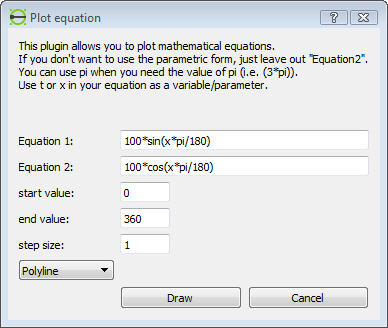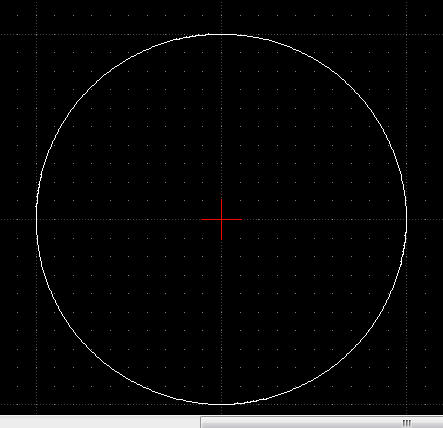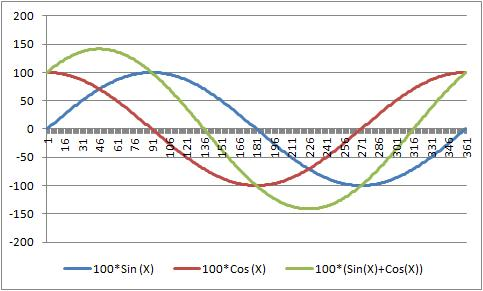All response will be highly appreciated. Best regard,
Open this post in threaded view
|

Re: Error Plotting Equation

 Please, if anyone can move this to Troubleshooting, please do it. I have unintentionally put this post in a wrong place. Best regard,
 Administrator I have no experience with this plugin, but after a look at the source code, I figured it out. You have to call the plugin for each curve with a single equation like this: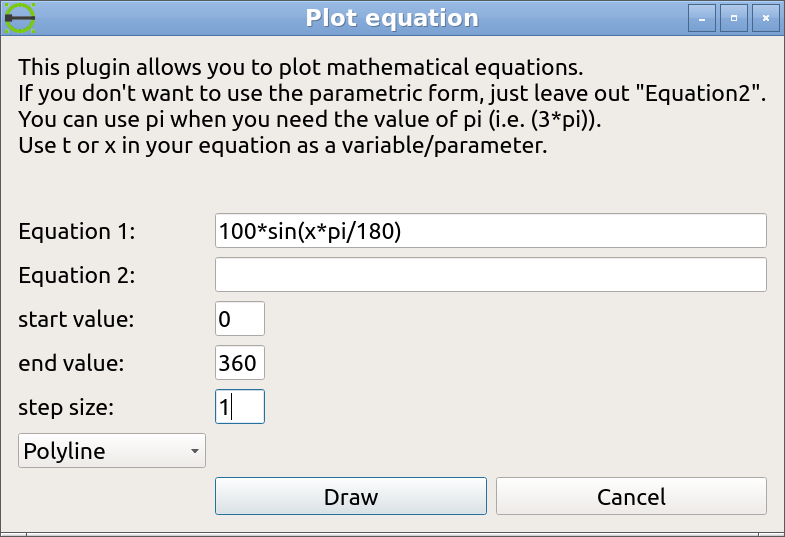Then use the cos() equation and the sin() + cos() equation with same start/end/step parameters. With two equations the first one is used for X values and the second for Y values. This explains your result, you have a circle with radius 100. Check this with start=0, end=180, this results in a half circle. Armin